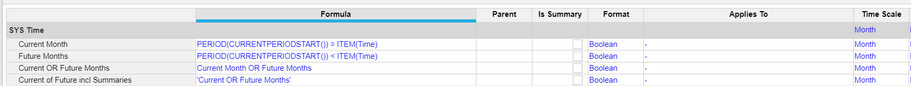# How I can made logic against time dimension?Hi,

I would like to understand how I can make a logic against Anaplan Time dimension list.

Target:

To make function for "Test" line item which tap True (to Test line item) for all periods where "Actual" current period is equal or smaller.

Cheers,

A

Tagged:

•Hi,

It depends on where you store "actual period".

If you store it into the Time tab then you can use the formula:

`CURRENTPERIODSTART()`

So to do what you want you could do:

IF START() <= CURRENTPERIODSTART() THEN TRUE ELSE FALSE

Actually to do extactly what you want, you'd better use CURRENTPERIODEND():

IF START() <= CURRENTPERIODEND() THEN TRUE ELSE FALSE

This formulas should be in your module called Time Filters. I advise that you take a look at the anaplan app where there is a lot of examples like that:

https://community.anaplan.com/t5/learning/Time-Filters/ba-p/28086

•If I want to put TRUE flag for all of those periods which earlier or same period than the current period (this is coming from time settings). This is my challenge.

•my answer was cut, take a look now
•You could reference the current period something similar to here:But it depends on what you want to achieve.

This allows you to identify future (or past) months. In essence every past month should have actuals (switchover)

All the best,

•For all time logic, keep the logic in a SYS Time module (only dimensionalized by Time) and then have your other modules reference this logic.  We see this all the time where people put if item(time) <=currentperiodstart() then .... in modules that are dimensioned by multiple which can lead to performance issues (logic being kicked off when it really doesn't need to).  @MaartenGijbels  has done this absolutely correct.

Rob

•